﻿ 纠错码在无线激光通信中的应用 The Application of Error-Correcting Code in the Wireless Laser Communication

Optoelectronics
Vol. 08  No. 04 ( 2018 ), Article ID: 27883 , 8 pages
10.12677/OE.2018.84024

The Application of Error-Correcting Code in the Wireless Laser Communication

Hengkuo Qu, Liang Yang, Jinli Tang, Yafeng Xie, Longchao Wei

The 27th Research Institute of CETC, Zhengzhou Henan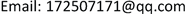Received: Nov. 19th, 2018; accepted: Nov. 29th, 2018; published: Dec. 6th, 2018ABSTRACT

Wireless laser communication refers to a technology of transmitting the information by laser carrier through the vacuum or atmosphere. In the laser communication the signal of receiver will become stronger or weaker, for falling under the atmosphere turbulence. So the error-correcting code was designed for the communication commonly. This article introduced the RS code, Turbo code and LDPC code. In the real system of wireless laser communication the RS code was used. At last of paper the result of experiment was showed between encoding and non-encoding. The result showed that the RS code can reduce the error-code-rate of system effectively.

Keywords:Wireless Laser Communication, Atmosphere Turbulence, Error-Correcting Code, RS CodeCopyright © 2018 by authors and Hans Publishers Inc.

This work is licensed under the Creative Commons Attribution International License (CC BY).

http://creativecommons.org/licenses/by/4.0/1. 引言

2. 大气湍流对激光通信的影响 

${\sigma }_{\beta }^{2}=\frac{1.093\cdot L\cdot {C}_{n}^{2}}{{D}^{1/3}}$

${R}_{\mathrm{max}_sg}=\frac{{R}_{\mathrm{max}_ss}}{\sqrt{1+{\sigma }_{\beta }^{2}{R}_{\mathrm{max}_ss}^{2}}}$

3. 纠错码技术  - 

3.1. RS码

RS码首先由Reed和Solomon于1960年构造出来，是目前最为有效、应用最为广泛的差错控制编码之一，是一类具有很强纠错能力的多进制BCH码，它不仅可以纠正突发错误，还可以纠正随机错误。

RS码的一个重要性质是：真正的最小距离总是相同的。每种RS码都是一个最大距离的可分码，最小距离等于n − k − 1。当省掉RS码的某些信息符号后，分组长度缩短，但是最小距离并不减小，故任何一种缩短的码仍是一个最大距离可分码。

RS码的另一个重要形式是在其码字内的任何k个位置都是可用信息集合。(n, k)RS码中，输入信息分成km比特一组，每组包括k个符号，每个符号由m比特组成。RS码的编译码是基于一组码元而不是单独的0或1，这也是RS码纠错能力特别强的原因，这也使得RS特别适合处理突发错误。

3.1.1. RS编码原理

RS(n, k)码具有以下性质：

n为码长，k为信息位长，n − k为校验位长。一般 $n=q-1$$n-k=2t$，q为有限域的阶数，是素数或素数的幂次，实用中一般取q为2的幂次。最小距离为 ${d}_{\mathrm{min}}=2t+1$，t为设计的纠错能力。RS码主要是围绕生成多项式构成的，其生成多项式为：

$\begin{array}{c}g\left(x\right)=\left(x-{\alpha }^{m}\right)\left(x-{\alpha }^{m+1}\right)\cdots \left(x-{\alpha }^{2t+m-1}\right)\\ ={a}_{n-k}{x}^{n-k}+{a}_{n-k-1}{x}^{n-k-1}+\cdots +{a}_{x}x+{a}_{0}\end{array}$

a为GF(q)域上本原多项式的根，a的各次幂可构成扩域GF(qm)上的所有元素。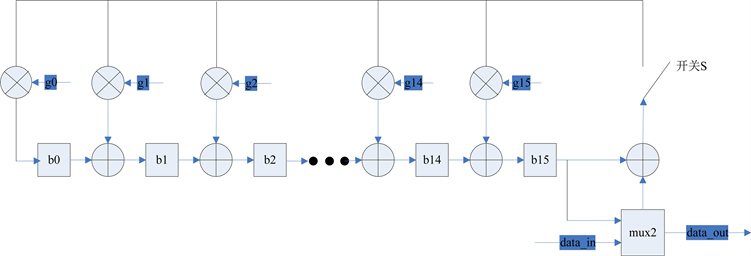Figure 1. The sketch map of encoding

3.1.2. RS码译码原理

RS译码分为以下几步：

1) 伴随式的求解

$g\left(x\right)=\left(x-{a}^{0}\right)\left(x-{a}^{1}\right)\cdots \left(x-{a}^{2t-1}\right)$

$H=\left[\begin{array}{ccccc}{\left({a}^{0}\right)}^{n-1}& {\left({a}^{0}\right)}^{n-2}& \cdots & {\left({a}^{0}\right)}^{1}& {\left({a}^{0}\right)}^{0}\\ {\left({a}^{1}\right)}^{n-1}& {\left({a}^{2}\right)}^{n-2}& \cdots & {\left({a}^{2}\right)}^{1}& {\left({a}^{2}\right)}^{0}\\ ⋮& ⋮& \ddots & ⋮& ⋮\\ {\left({a}^{2t-1}\right)}^{n-1}& {\left({a}^{2t-1}\right)}^{n-2}& \cdots & {\left({a}^{2t-1}\right)}^{1}& {\left({a}^{2t-1}\right)}^{0}\end{array}\right]$

${S}_{j}=\underset{i=1}{\overset{t}{\sum }}{E}_{{l}_{i}}{x}_{i}^{j-1},\text{\hspace{0.17em}}\text{\hspace{0.17em}}j=1,2,\cdots ,2t$

2) 差错位置多项式的确定

$\begin{array}{c}\sigma \left(x\right)=\left(1-{x}_{1}x\right)\left(1-{x}_{2}x\right)\cdots \left(1-{x}_{t}x\right)\\ =1+{\sigma }_{1}x+{\sigma }_{2}{x}^{2}+\cdots +{\sigma }_{t}{x}^{t}\end{array}$

${x}_{1}^{\sigma }$${x}_{2}^{\sigma }$，L， ${x}_{t}^{\sigma }$ 是σ(x)的解，由σ(x)的定义有

${x}_{1}={\left({x}_{1}^{\sigma }\right)}^{-1},{x}_{2}={\left({x}_{2}^{\sigma }\right)}^{-1},\cdots ,{x}_{t}={\left({x}_{t}^{\sigma }\right)}^{-1}$

3) 关键方程的求解

ME迭代算法可以简单描述为以下几个公式和约束条件的迭代。首先初始化： ${R}_{0}\left(x\right)={x}^{2t}$${Q}_{0}\left(x\right)=S\left(x\right)$${L}_{0}\left(x\right)=0$${U}_{0}\left(x\right)=1$；迭代后如果 $\mathrm{deg}\left({R}_{i}\left(x\right)\right)$$\mathrm{deg}\left({Q}_{i}\left(x\right)\right)$ 小于8，则算法迭代结束。此时 $\omega \left(x\right)={R}_{i}\left(x\right)$$\sigma \left(x\right)={L}_{i}\left(x\right)$

4) 错误位置的确定

$R\left(x\right)={r}_{n-1}{x}^{n-1}+{r}_{n-2}{x}^{n-2}+\cdots +{r}_{1}x+{r}_{0}$

$\sigma \left({\alpha }^{-\left(n-1\right)}\right)=\sigma \left(\alpha \right)=1+{\sigma }_{1}\alpha +{\sigma }_{2}{\alpha }^{2}+\cdots +{\sigma }_{t}{\alpha }^{t}=0$

5) 差错幅值的求解

${s}_{t}=\underset{k=1}{\overset{7}{\sum }}{Y}_{k}{x}_{k}^{i}$

$-{\sigma }^{\prime }\left({x}_{i}^{-1}\right){x}_{i}^{-1}=\underset{\begin{array}{l}j=1\\ j\ne i\end{array}}{\overset{\gamma }{\prod }}\left(1-{x}_{j}{x}^{-1}\right)$，同时得到

${Y}_{i}{x}_{i}{x}_{i}^{-1}\left(-{\sigma }^{\prime }\left({x}_{i}^{-1}\right)\right)\left({x}_{i}^{-1}\right)=\omega \left({x}_{i}^{-1}\right)$

3.2. Turbo码

Turbo码是C. Berrou等人提出了一种新型的纠错码。它采用一种并行级联的方法实现了长码的编码，同时构造了相应的解码器来完成长码的解码，是一种实用的纠错码，它的性能非常逼近香农的性能界，使其在较低的信噪比条件下仍能够保持良好的性能。目前Turbo码在CDMA的应用得到各国学者的重视，Turbo迭代的思想已经渗透到物理链路层先进的信号处理技术的各个领域，它域调制、均衡、空时编码和OFDM相结合极大的促进了技术的进步和发展。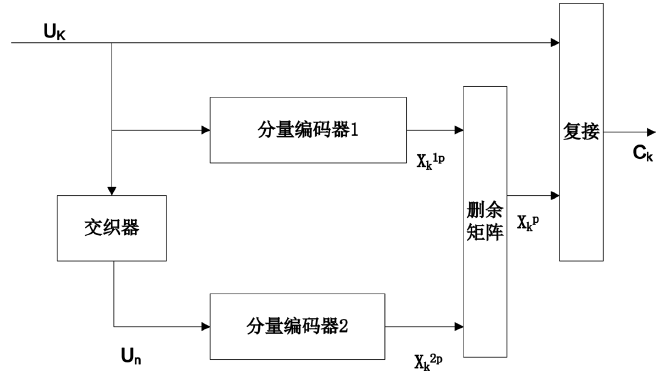Figure 2. The sketch map of turbo encoding

3.3. LDPC(低密度奇偶校验)码

LDPC码是Gallager于1962年提出的一种性能接近于香农限的好码，限于当时计算机水平和硬件技术LDPC码在很长一段时间内一直未受到人们重视，直到Berrou等人提出Turbo码后，LDPC码才重新引起人们的研究兴趣并成为研究的热点。计算机仿真结果表明，最好的非规则LDPC码(长度为106)可获得在BER = 10−6时仅偏离容量0.13 dB的性能，优于迄今所知道的最佳Turbo码，当码长为107码率为1/2时，其性能距香农限只差0.04 dB。

LDPC码的特点是：性能优于Turbo码，具有较大灵活性和较低的差错平底特性；译码复杂度低于Turbo码，且可实现完全的并行操作，在编码器和译码器之间不需要交织器，硬件复杂度低，因而适合硬件实现；吞吐量大，适合高码率的应用，极具高速译码潜力。此外，LDPC码在干扰、衰落等原因引起的突发误码情况下有更优良的性能。但LDPC码在硬件实现上难点是它的不规则结构，大部分的LDPC码的结构都是非常随机的，使得它的编码器要比Turbo码的复杂一些。另一个缺点是不能灵活地改变码率和码长，要改变通常需要重新构造奇偶校验矩阵和相应地生成矩阵，使得硬件改动困难。

4. RS码在无线激光通信链路中的应用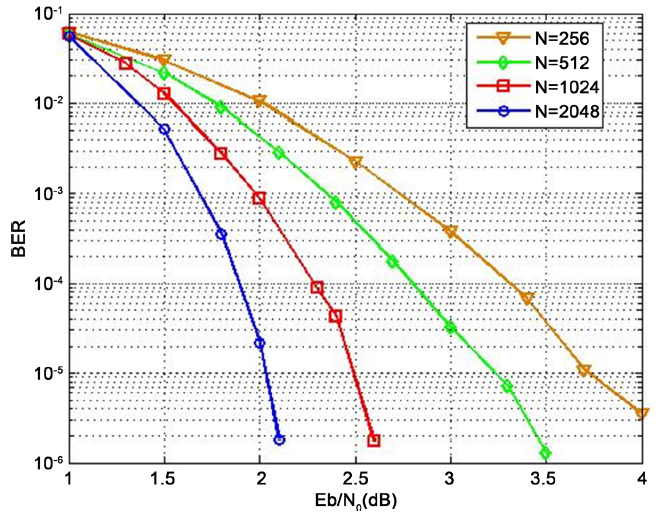Figure 3. The compare of difference frame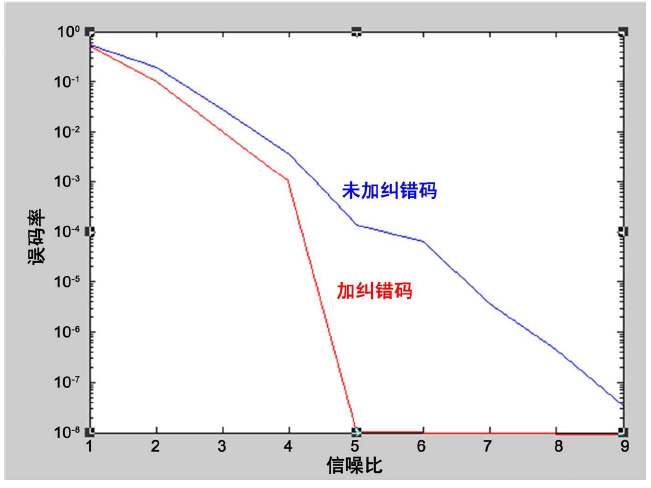Figure 4. The error-code-rate between encoding and nun encoding

5. 结论

The Application of Error-Correcting Code in the Wireless Laser Communication[J]. 光电子, 2018, 08(04): 184-191. https://doi.org/10.12677/OE.2018.84024

1. 1. 饶瑞中. 光在湍流大气中的传输[M]. 合肥: 安徽科学技术出版社, 2005.

2. 2. 强世锦, 荣健. 基于无线激光通信仿真系统的纠错码性能比较[J]. 光通信研究, 2008(3): 17-18.

3. 3. 何晓垒, 熊汉林, 雷利娟. 基于无线激光通信系统的FEC应用研究[J]. 信息通信, 2010(4): 36-38.

4. 4. 张吾进, 毛倩. 基于Turbo码的纠错加密联合编码方案[J]. 无线电通信技术, 2012, 38(5): 29-32.

5. 5. 高雨明, 费强. 前向纠错码技术分析及其应用[J]. 邮电设计技术, 2010(9): 63-66.

6. 6. 徐建武, 王红星, 胡昊. Turbo乘积码技术及其性能研究[J]. 现代电子技术, 2008(15): 21-26.

7. 7. 雷婷, 张建志. LDPC编译码算法分析[J]. 无线电工程, 2012, 42(10): 8-10.

8. 8. 贾科军, 柯熙政, 彭铎, 陈海燕. 无线激光通信系统中π旋转LDPC码的设计与性能分析[J]. 兰州理工大学学报, 2008, 34(4): 109-103.

9. 9. 赵旸, 耿相铭. RS(16,12)缩短码编译码原理及性能分析[J]. 通信技术, 2012, 45(2): 49-52.